# 机器学习-NLP之Word embedding 原理及应用

2019/04/10 10:10

• 概述

• One-hot encoding Word Representation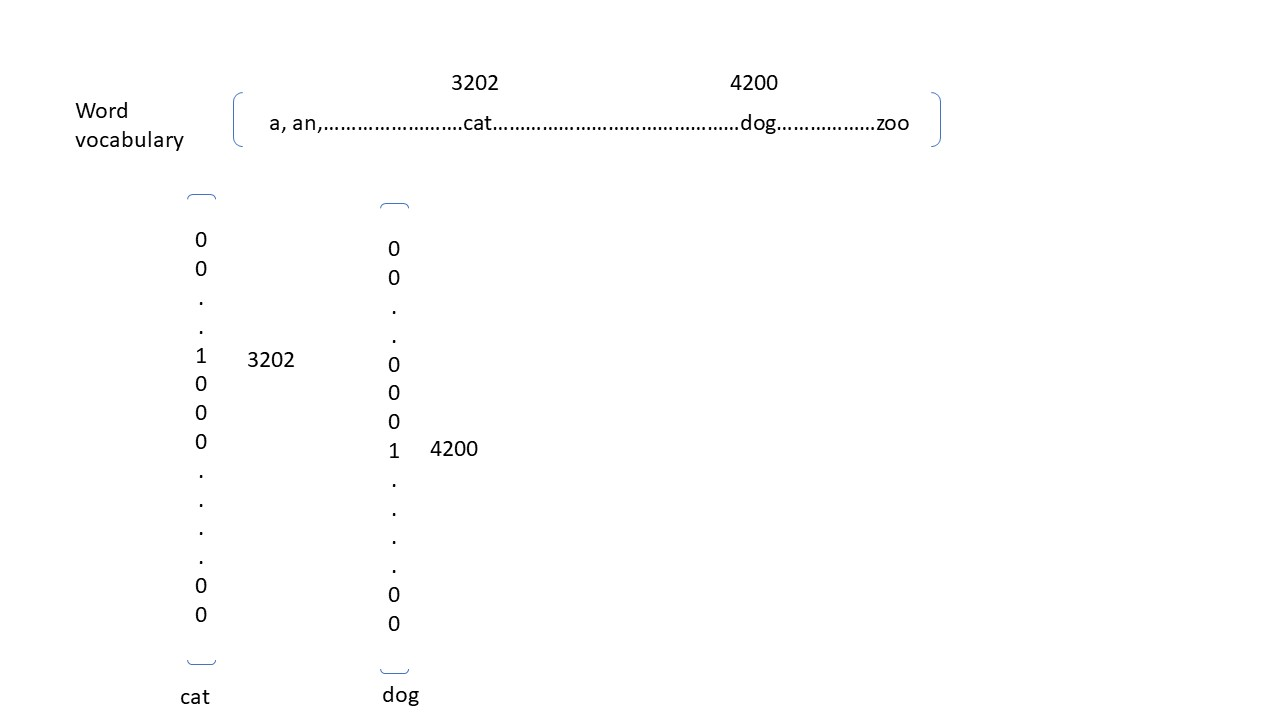•  Featured representation - word embedding

Word embedding 应该是咱们整个NLP中最最常用的一个技术点了，它的核心思想就是每一个Word都有一些维度，具体有多少维度咱们用户自己定义，但是这些维度能保证相似的词汇在这个多维的空间上的值是相似的，例如咱们用5维的空间来表示一个词，那么cat可能是[1.0, 1.5, 0.0, 6.4, 0.0], dog可能是[0.95, 1.2, 0.11, 5.5, 0.0], book可能的值是[9.5, 0.0, 3.4, 0.3, 6.2]。从前面的几个值咱们可以很显然的看出cat和dog在这个五维空间是比较接近的，而他们跟book则在这个五维空间上的距离要远的多，这也更加符合咱们的实际情况的认知，那么具体这个五维空间的每一个feature是什么，咱们不需要知道，在后面的部分我会介绍2红算法来计算embedding的。那么下面就接着上面的例子，我画一个图片更加方便大家的理解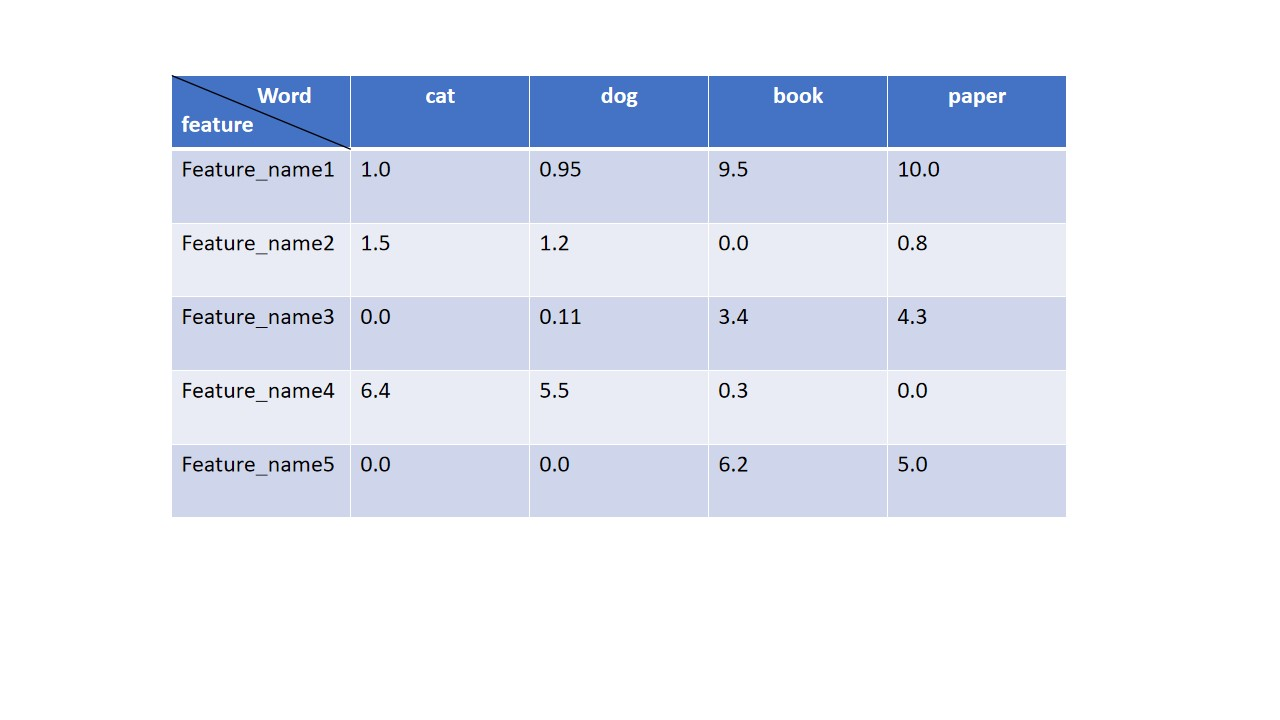• Embedding - Neural Network NLP modeling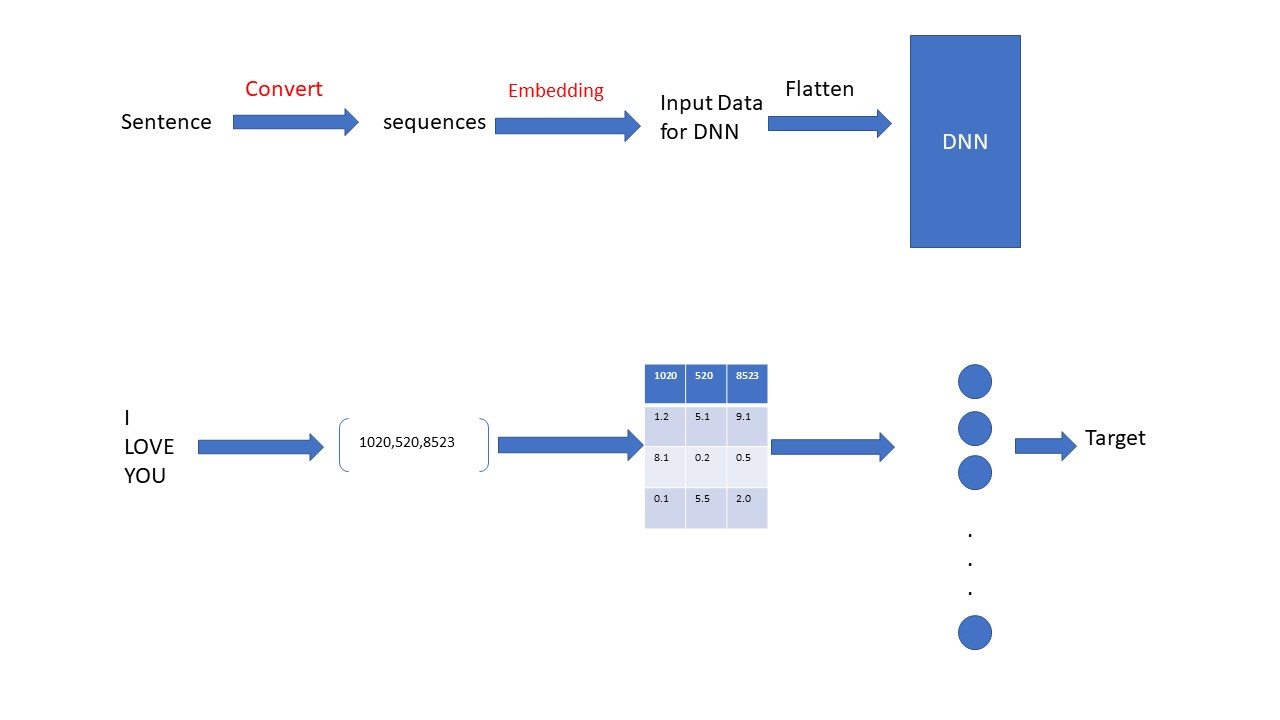•  Embedding - Skip-Grams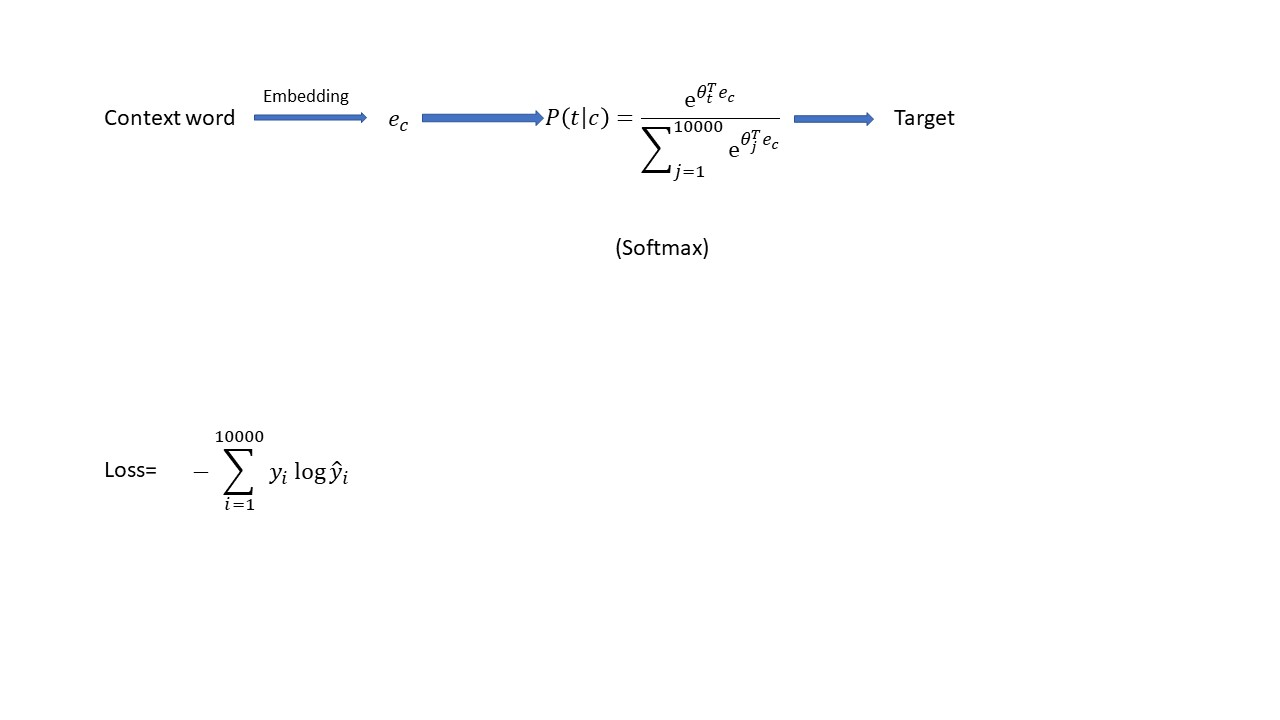•  TensorFlow应用之Embedding （Text sentiment analysis）

``````import tensorflow as tf
import tensorflow_datasets as tfds
import numpy as np

train_data = imdb["train"]
test_data = imdb["test"]``````

``````training_sentences = []
training_labels = []
test_sentences = []
test_labels = []

for s,l in train_data:
training_sentences.append(str(s.numpy()))
training_labels.append(l.numpy())

for s,l in test_data:
test_sentences.append(str(s.numpy()))
test_labels.append(l.numpy())``````

``````from tensorflow.keras.preprocessing.text import Tokenizer

max_length = 120
trunc_type="post"
oov_tok = "<OOV>"

#initialize a tokenizer
tokenizer = Tokenizer(num_words = vocab_size,oov_token = oov_tok)
#fit the text to create word index, result a dict of the word:index key-values
tokenizer.fit_on_texts(training_sentences)
word_index = tokenizer.word_index

#create a sequences of the training sentences based on the dictionary above(word-index)
training_sequences = tokenizer.texts_to_sequences(training_sentences)

#create a sequences of the test sentences based on the dictionary above(word-index)
test_sequences = tokenizer.texts_to_sequences(test_sentences)

``````vocab_size = 10000
embedding_dim = 16

#define model structure
model = tf.keras.Sequential([

tf.keras.layers.Embedding(vocab_size,embedding_dim,input_length=max_length),
tf.keras.layers.Flatten(),
tf.keras.layers.Dense(64,activation="relu"),
tf.keras.layers.Dense(1,activation="sigmoid")

])
model.summary()``````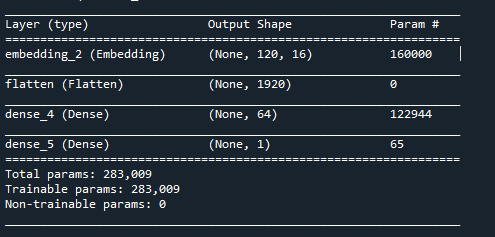上面可以完整的看到咱们定义的网络结构和一些参数。

``````#training the model
model.fit(
training_labels_final,
epochs=10,
)``````

0
0 收藏

### 作者的其它热门文章0 评论
0 收藏
0